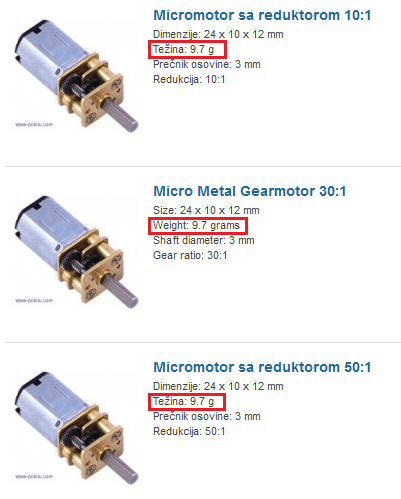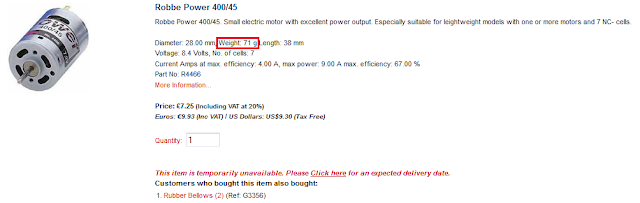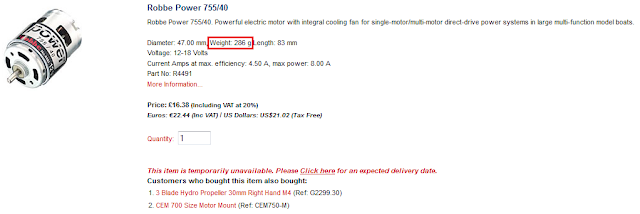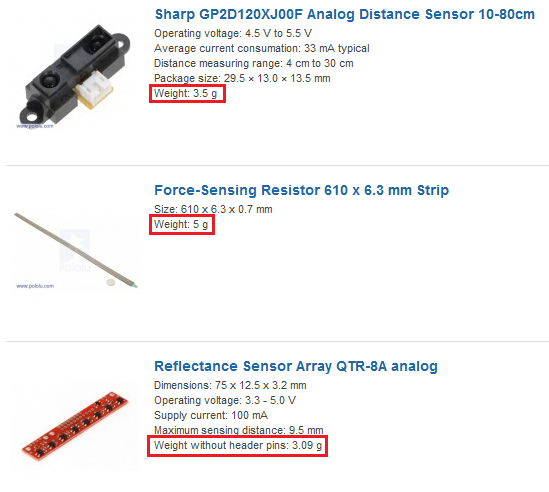### Normal force - Real catalog examples and calculations

We already started with articles about mobile robot fundamentals and Normal force which play important role in mobile robot hardware development. Links to related articles are available at the end of this article, but let’s proceed with some real catalog examples and calculations regarding normal force.

The mobile robot regarding construction is nothing else than a composite mass, composed of several different physical components. Each physical component has its mass, which also mean that it has weight. When mass interacts with the surface, reactional force called Normal force is generated by the ground surface, acting on the center of the mass. For example, if mobile robot is composed of six physical components, total weight of the mobile robot is a summary of all six physical components together, with only one center of the mass. Let’s start with real catalog examples and calculations.Figure #1. Catalog information was taken from rcsrbija.rs

Figure #1 shows a catalog representation of three DC motors equipped with gearboxes. As you can see, beside parameters like: size, gear ratio, shaft diameter there is also information about weight. Unfortunately the unit of measure regarding weight is set to gram instead of Newton. Generally, almost in every catalog, you will see gram as the weight unit of measure, even if we do know that weight is a force which means that unit of measure is Newton. Now, let’s say that we are building a differential mobile robot composed of two DC motors. We already made a selection of DC motor, for example, Micro Metal Gearmotor 30:1. By catalog information, weight of the Micro Metal Gearmotor is 9.7 grams. Because we need two DC motors, we have 9.7 g x 2 = 19.4 grams in total. But that is only weight of DC motors. Total weight of mobile robot as we already said in this article include weight of other physical components like: sensor, wheels, chassis etc.Figure #2. Catalog information was taken from www.cornwallmodelboats.co.uk/acatalog/Robbe-Motors.html

Figure #2 shows a catalog representation of the Robbe Navy S 4.8V DC motor. In comparison with Micro Metal DC motor, Robbe is not equipped with gearbox, but it has mounting holes for gearbox installation, please pay attention on mounting holes right beside the DC motor shaft (front end of DC motor chassis). But that is not only difference. Weight is another difference and for Robbe Navy it is a 42 grams. For differential mobile robot we need two DC motors, so if we choose Robbe Navy for our mobile robot, total weight regarding DC motors is 42 x 2 = 84 grams.Figure #3. Catalog information was taken from www.cornwallmodelboats.co.uk/acatalog/Robbe-Motors.html

Figure #3 shows a catalog representation of the Robbe Power 400/45 DC motor. Its weight is 71 grams. If we choose Robbe Power 400, total weight regarding DC motors is 71 x 2 = 142 grams.Figure #4. Catalog information was taken from www.cornwallmodelboats.co.uk/acatalog/Robbe-Motors.html

Figure #4 shows a catalog representation of the Robbe Power 755/45 DC motor. Its weight is 286 grams. If we choose Robbe Power 755, total weight regarding DC motors is 286 x 2 = 572 grams. Beside weight, catalog data provide information about supply voltage and current consumption. Current consumption is important parameter regarding DC motor driver selection, power transistors selection, etc. Fundamentals regarding DC motor drivers are covered in the following article Programmable autonomous vehicles – Fundamentals, Part I.Figure #5. Catalog information was taken from rcsrbija.rs

Figure #5 shows a catalog representation regarding three different sensing devices. Sharp GP2D120XJ00F analog distance sensor is one very popular sensor commonly used by mobile robot's developers. Beside parameters like: operational voltage, distance measuring range, etc., catalog present information about sensor weight. Sharp analog sensor weight is 3.5 grams. Let’s say that our mobile robot is equipped with five distances sensing devices and two force sensing resistors. In this case, weight is: (3.5 grams x 5) + (5 grams x 2) = 17.5 grams + 10 grams = 27.5 grams in total.Figure #6. Catalog information was taken from rcsrbija.rs

Figure #6 shows a catalog representation regarding mobile robot wheels. Beside dimension, weight is also presented as parameter. For differential mobile robot we do need two of these. In that case weight is: 3.2 grams x 2 = 6.4 grams.

Now let’s do some quick example, calculations regarding following parameters:

- Mobile robot chassis weight is 350 grams
- Control unit – microcontroller board weight is 25 grams
- DC motor driver weight is 250 grams
- 2x Robbe Power 755/45 DC motor weight is 572 grams
- Sensing devices total weight is 27.5 grams
- 2 x wheel, total weight is 6.4 grams

In total, mobile robot weight is: 350 grams + 250 grams + 25 grams + 572 grams + 27.5 grams + 6.4 grams = 1230.9 grams. Calculated weight is the total weight of mobile robot closely related to Normal force as it is already discussed in related article Feel the forcearound you – Normal force.

Related articles:
Feel the force around you – Normal force
Feel the force around you – Frictional force Part I
Feel the force around you – Frictional force Part II
Feel the force around you – Frictional force Part III
Feel the force around you – Frictional force Part IV

Programmable autonomous vehicles – Fundamentals, Part I